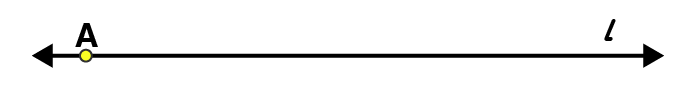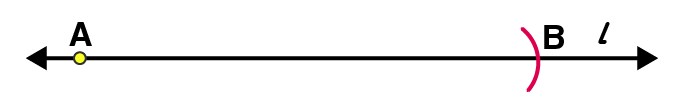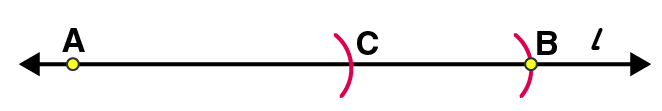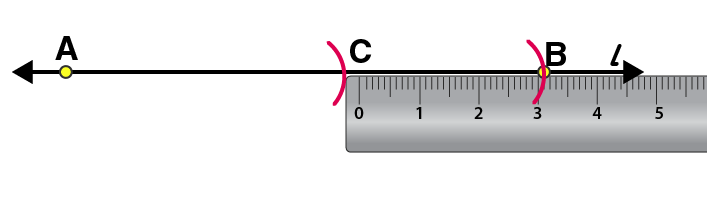# Construct $$\overline{AB}$$. From this, cut off $$\overline{AC}$$ of length 4.7 cm. Measure $$\overline{BC}$$

Solution:

Step 1: Draw a line l and mark a point A on it.Step 2: By adjusting the compasses up to 7.8 cm, while placing the pointer of compasses on point A draw an arc to cut l on B $$\overline{AB}$$ is the line segment of 7.8 cmStep 3: By adjusting the compasses up to 4.7 cm, draw an arc to cut l on C, while placing the pointer of compasses on point A. $$\overline{AC}$$ is the line segment of 4.7 cmStep 4: Now, place the ruler in such a way that the 0 mark of the ruler will match with point C.Now read the position of point B. It will be 3.1 cm. $$\overline{BC}$$ is 3.1 cm(0)(0)